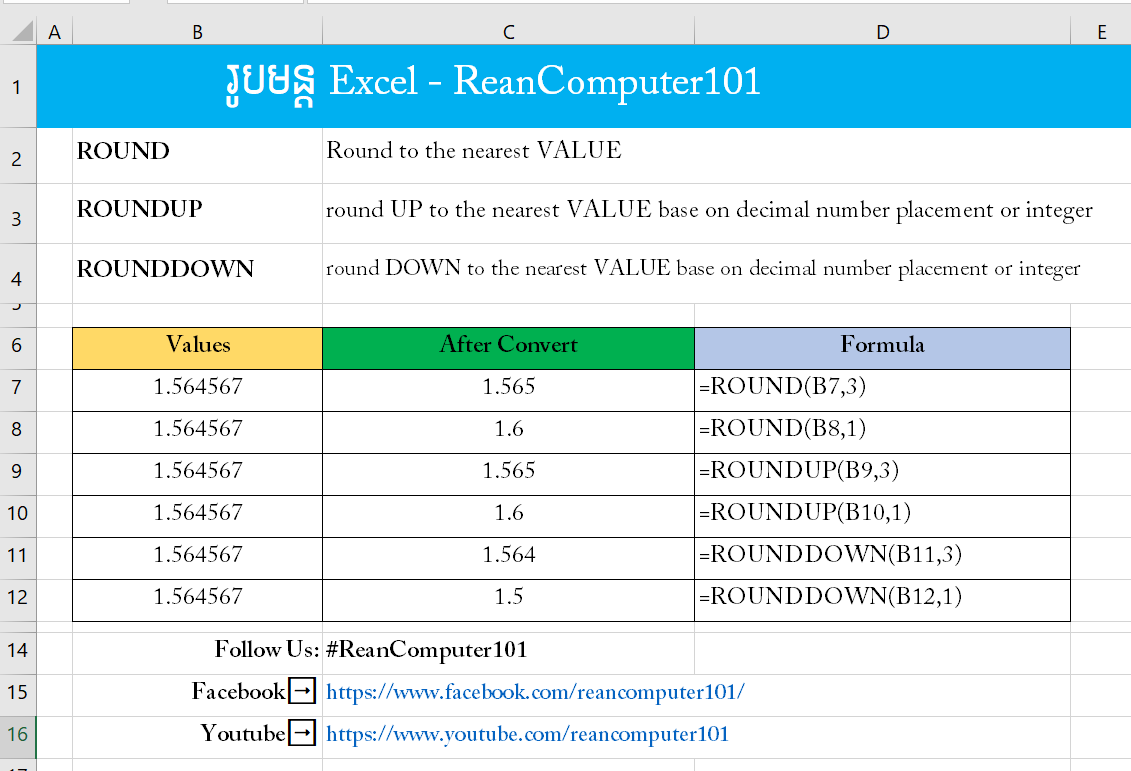## How to use ROUND, ROUNDUP and ROUNDDOWN formula in Excel with Example Download

Convert value in Microsoft EXCEL sometime so complicated. This tutorial shows how to round your value up or down with ROUND, ROUNDDOWN and ROUNDUP formulas in Excel. Refer to video for more detail.
For example:
Value 1.564567 to 1.6 or 1.5### ROUND formula in Excel

ROUND returns the nearest value by specify digit number. It can be up or down depend on value and num_digits paramatter.
FORMULA:
=ROUND(number, num_digits)
◾ number: Required, number you want to round.
◾ num_digits: required, number of digit you want to round value to.

### ROUNDDOWN formula in Excel

ROUNDDOWN rounds a number down, toward zero.
FORMULA:
=ROUNDDOWN(number, num_digits)
◾ number: Required, any number want want to round down.
◾ num_digits: required, number of digit you want to round value to.

### ROUNDUP formula in Excel

ROUNDUP rounds a number up, away from zero.
FORMULA:
=ROUNDUP(number, num_digits)
◾ number: Required, any number want want to round up.
◾ num_digits: required, number of digit you want to round value to.
Watch How to use ROUND, ROUNDUP and ROUNDDOWN formula in Excel speak Khmer